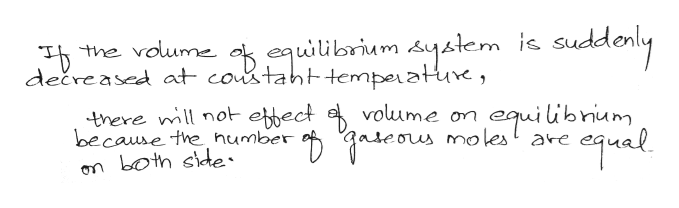# Consider the following system at equilibrium where H° = 18.8 kJ, and Kc = 9.52×10-2, at 350 K.CH4(g) + CCl4(g) 2CH2Cl2(g)If the VOLUME of the equilibrium system is suddenly decreased at constant temperature:The value of Kc A. increases.  B. decreases.  C. remains the same. The value of Qc A. is greater than Kc.  B. is equal to Kc.  C. is less than Kc. The reaction must: A. run in the forward direction to reestablish equilibrium.  B. run in the reverse direction to reestablish equilibrium.  C. remain the same. It is already at equilibrium. The number of moles of CCl4 will: A. increase.  B. decrease.  C. remain the same.

Question
68 views

Consider the following system at equilibrium where H° = 18.8 kJ, and Kc = 9.52×10-2, at 350 K.

CH4(g) + CCl4(g) 2CH2Cl2(g)

If the VOLUME of the equilibrium system is suddenly decreased at constant temperature:

 The value of Kc A. increases. B. decreases. C. remains the same.

 The value of Qc A. is greater than Kc. B. is equal to Kc. C. is less than Kc.

 The reaction must: A. run in the forward direction to reestablish equilibrium. B. run in the reverse direction to reestablish equilibrium. C. remain the same. It is already at equilibrium.

 The number of moles of CCl4 will: A. increase. B. decrease. C. remain the same.
check_circle

Step 1
Step 2help_outlineImage Transcriptionclosethe voume k equiibrium system dečreased at costaht tempeatue, uddonly is sud there mll not ebect because the humber on both side volume on w molest are equilibniam fullscreen

### Want to see the full answer?

See Solution

#### Want to see this answer and more?

Solutions are written by subject experts who are available 24/7. Questions are typically answered within 1 hour.*

See Solution
*Response times may vary by subject and question.
Tagged in

### Equilibrium Concepts